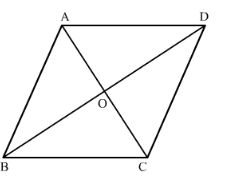# Each side of a rhombus is 10 cm. If one its diagonals is 16 cm find the length of the other diagonal.

Question:

Each side of a rhombus is 10 cm. If one its diagonals is 16 cm find the length of the other diagonal.

Solution:

We know that a quadrilateral is said to a rhombus if all sides of the quadrilateral are equal. Diagonals of a rhombus bisect each other at right angles.

Quadrilateral ABCD is a rhombus and diagonals AC and BD intersect at point O.As we defined above, we get $\mathrm{AB}=\mathrm{BC}=\mathrm{CD}=\mathrm{AD}, \mathrm{AO}=\mathrm{OC}, \mathrm{BO}=\mathrm{OD}$ and angle $\angle \mathrm{AOD}=\angle \mathrm{AOB}=\angle B O C=\angle C O D=90^{\circ}$.

We are given that AB = 10 cm and AC = 16 cm. Now we will find length of BD.

As we know the definition of rhombus we get AB = BC = CD = AD.

Therefore, we get, AB = BC = CD = AD = 10 cm

Also we know that diagonals of rhombus bisect each other at right angles therefore, we get,

$\mathrm{AO}=\mathrm{OC}$

$\mathrm{BO}=\mathrm{OD}$

and $\angle \mathrm{AOD}=\angle \mathrm{AOB}=\angle B O C=\angle C O D=90^{\circ}$

Here, we know the length of $A C$ therefore, we get, $A O=O C=8 \mathrm{~cm}$.

Now we will use Pythagoras theorem in the right angled triangle AOD as below,

$\mathrm{AD}^{2}=\mathrm{AO}^{2}+\mathrm{OD}^{2}$.....(1)

Now we will substitute the values of AD and AO in equation (1) we get,

$10^{2}=8^{2}+O D^{2}$

$100=64+O D^{2}$...(2)

Now we will subtract 64 from both sides of the equation (2)

$O D^{2}=100-64$

$O D^{2}=36$

Now we will take the square root.

OD = 6

We know that $B D=2 O D$

$\therefore B D=2 \times 6$

$\therefore B D=12$

Therefore, length of the other diagonal is $12 \mathrm{~cm}$.